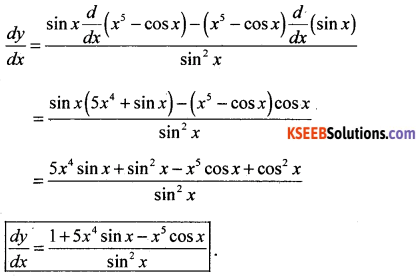# 1st PUC Maths Previous Year Question Paper March 2019(North)

Students can Download 1st PUC Maths Previous Year Question Paper March 2019, Karnataka 1st PUC Maths Model Question Papers with Answers helps you to revise the complete Karnataka State Board Syllabus and score more marks in your examinations.

## Karnataka 1st PUC Maths Previous Year Question Paper March 2019(North)

Part-A

Answer the following questions (10 × 1 = 10 )

Question 1.
Write the set A = {x : x is a natural number less than 6} in roaster form
B = {1,2,3,4,5 }

Question 2.
If P = {a, b, c) and Q = {r}, find P × Q.
P × Q = {(a,r),(b,r),(e,r)}

Question 3.
Convert $$\frac{7 \pi}{6}$$ radians in degree measure.
210°

Question 4.
Express (-5 i) ($$\frac { 1 }{ 8 }$$ i) in the form a + ib.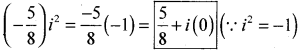Question 5.
Evaluate 4! – 3!
4! – 3! = (4 × 3 × 2 × 1) – (3 × 2 × 1) = 24 – 6 = 18

Question 6.
Write the first three terms of the sequence defined by an = 2n + 5
an = 2n + 5; a1 = 7, a2 = 9, a3 = 11

Question 7.
Find the slope of the line passing through the points (3, -2) and (-1, 4).Question 8.
Evaluate $$\lim _{x \rightarrow 4}\left(\frac{4 x+3}{x-2}\right)$$
$$=\frac{4(4)+3}{4-2}=\left[\frac{19}{2}\right]$$

Question 9.
Write the negation of the statement ‘Australia is a continent’.
Australia is not a continent.

Question 10.
A coin is tossed three times. What is the sample space for the experiment?Part – B

Answer any TEN questions: (10 × 2 = 20)

Question 11.
If A = {3, 5, 7, 9, 11}, B = {7, 9, 11, 13} and C = {11, 13, 153. Find A ∩ (B ∪ C).
A ∩ (B ∪ C) = {3,5,7,9,11} ∩ {7,9,11,13,15} = {7,9,11}

Question 12.
If x and y are two sets such that n(x) = 17, n(y) = 23 and n(x ∪ y) = 35, find n(x ∩ y)
n(X ∩ Y) = n(X) + n(Y) – n(X ∪ Y) = 17 + 23 – 38 = 2.Question 13.
Let f = {(1,1), (2,3), (0, -1), (-1, -3) be a linear function f(x)= mx + c from Z into Z. Find the value of m and c.
Let the function be f(x) = mx + c, f(0) = -1 = c = -1
f(1) = m. 1 + c ⇒ 1 = m – 1 ⇒ m = 2 ∴ f(x) = 2x – 1

Question 14.
Find the radius of the circle in which a central angle of 60° intercepts an arc of length 37.4 cm (given π = $$\frac{22}{7}$$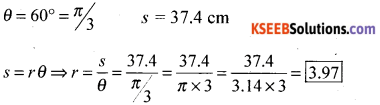Question 15.
Find the general solution of cos x = $$\frac { 1 }{ 2 }$$
cos x = $$\frac { 1 }{ 2 }$$, General solution : x = 2nπ ± $$\frac{\pi}{3}$$

Question 16.
Solver x2 + x + 1 = 0Question 17.
The marks obtained by a student of class Xl in the first and second terminal examination are 62 and 48 respectively. Find the minimum marks he should get in the annual examination to have an average of at least 60 marks.
Let ‘x’ be the marks obtained by student.
Then $$\frac{62+48+x}{3} \geq 60$$ ⇒ 110x + x ≥ 180, x ≥ 70

Question 18.
Find the equation of the line passing through the point (-2, 3) and having slope – 4.
y – 3 = -4 (x + 2)
i.e., 4x + y + 5 = 0.

Question 19.
Find the distance of the point (3, -5) from the line 3x – 4y – 26 = 0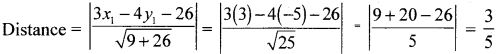Question 20.
Find the co-ordinates of the point P which divides the line segment joining the points and B (3, 4, -5) internally in the ratio 2:3.Question 21.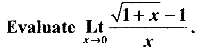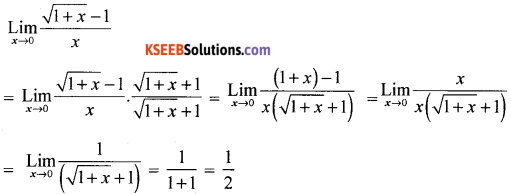Question 22.
Write the component statement of the following compound statement and check whether they are true or false “All prime numbers are either even or odd’.
Converse
If x is odd then x is prime

Contrapositive
If x is not odd then x is not prime.Question 23.
Two series A and B with equal means have standard deviation 9 and 10 respectively. Which series is more consistet?Question 24.
A committe of two persons is selected from two men and two women. what is the probability that the committee will have a) no man b) one man.
The total number of persons = 2 + 2 = 4. Out of these four person, two can be selected in 4C2 ways.
(a) No men in the committee of two means there will be two women in the committee.
Out of two women, two can be selected in 2C2 = 1 way.
Therefore P(no man) = $$\frac{^{2} C_{2}}{^{4} C_{2}}=\frac{1 \times 2 \times 1}{4 \times 3}=\frac{1}{6}$$

(b) Two men can be selected in 2C2 ways.
Hence P(Two man) = $$\frac{^{2} \mathrm{C}_{2}}{^{4} \mathrm{C}_{2}}=\frac{1}{^{4} \mathrm{C}_{2}}=\frac{1}{6}$$

Part – C

Answer any TEN questions: (10 × 3 = 30)

Question 25.
Let u = (1, 2, 3, 4, 5, 6), A = {2,3} and B = {3,4,5}. show that (A ∪ B)’ = A’ ∩ B’
A ∪ B = {2,3,4,5} (A ∪ B)’ = {1,6}
A’ = {1,4,5,6} B’= {1,2,6}
A’ ∩ B’ = {1,6}
∴ (A ∪B)’ = A’ ∩ B’

Question 26.
Let f(x)= x2, g(x) = 2x + 1 be two real functions. Find
(i) (f + g) (x) (ii) (f – g)(x) (iii) (f.g) (x)
(i) f + g(x) = f(x) + g(x) = x2 + 2x + 1
(ii) (4 – 8)(x) = f (x) – g(x) = x2 – 2x – 1
(iii) (f × g)(n) = f(x) × g(x) = x2 (2x + 1) = 2x3 + x2

Question 27.
Prove that tan3x = $$\frac{3 \tan x-\tan ^{3} x}{1-3 \tan ^{2} x}$$
We have tan 3x = tan (2x + x)Question 28.
Represent the complex number Z = 1 + i√3 in the polar form.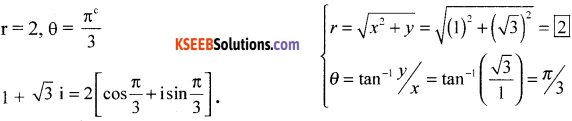Question 29.
If x + iy = $$\frac{a+i b}{a-i b}$$, prove that x2 + y2 = 1
we have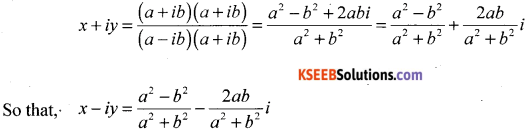Therefore,Question 30.
In how many of the distinct permutations of the letters in ‘MISSISSIPPI do the four I’s e not come together.
The given word has 11 letters out of which there are four I’s, four S’s, two P’s and one M.
∴ total number of permutations = $$\frac{11 !}{4 ! 4 ! 2 !}$$
Now consider four I’s as one letter and 7 others which are fourS’s two P’s and one M. So the number of permutations in which four I’s are together = $$\frac{8 !}{4 ! \times 2 !}$$
∴ the number of permutations in which the four I’s do not come together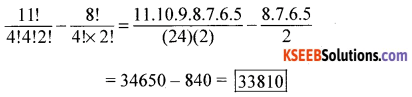Question 31.
Find the term independent of x in the expansion of $$\left(\frac{3 x^{2}}{2}-\frac{1}{3 x}\right)^{6}$$
We have TThe term will be independent of x if the index of x is zero i.e., 12 – 3r = 0. Thus, r= 4
Hence 5th term is independent of x and is given (-1)46C4 $$\frac{(3)^{6-8}}{(2)^{6-4}}=\frac{5}{12}$$

Question 32.
In an A.P if mth term is n and nth is m, where m ≠ n, find the pth term .
am = a + (m – 1)d = n …..(1)
an = a + (n – 1)d = m …..(2)
Solving (1) and (2) we get
(m – n)d = n – m
∴ d = -1
& a = m + n – 1
Now ap = a + (p – 1)d = (m + n – 1) + (p – 1)(-1)
ap = m + n – p

Question 33.
Find the sum of the sequence 8, 88, 888, 8888, … to n terms.
Sn = 8 + 88 + 888 + ………………
= 8[1 + 11 + 111 + ………….. ]
= $$\frac{8}{9}$$(9 + 99 + 999 + ……nth term) = $$\frac{8}{9}$$[(10 – 1)+(102 – 1]+ ………]
= $$\frac{8}{9}$$[10(102 – 1) ] [(10 + 102 + 103 + ……. nth term) – (1 + 1 + 1 + …..)] = $$\frac{8}{9}\left[\frac{10\left(10^{\mathrm{n}}-1\right)}{(10-1)}-\mathrm{n}\right]$$

Question 34.
Find the centre and radius of the circle x2 + y2 + 8x + 10y – 8 = 0
x2 + y2 + 8x + 10y – 8 = 0 ⇒ (x2 + 2.x.4 + 16) -16 + (y2 + 2.y.5 + 25) – 25 – 8 = 0
⇒ (x + 4)2 + (y + 5)2 = 49 ⇒ centre = (-4,-5), radius = 7

Question 35.
Find the derivative of cos x from first principle.
Let f(x) = cos x. ∴ f(x + Δx) = cos(x + Δx).Question 36.
Verify by the method of contradiction that “√7 is irrational”.”
In this method, we assume that the given statement is false i.e., we assume that √7 is rational.
∴ there exist positive integers a and b such that √7 = $$\frac { a }{ b }$$ ,where a and b have no common factors. Squaring the equation, we get 7 = $$\frac{a^{2}}{b^{2}}$$ ⇒ a2 = 7b2 ⇒ 7 divides ‘a’.
∴ there exists an integer ‘e’ such that a = 7c ⇒ a2 = 49c2
Now a2 = 49c2 and a2 = 7b2 ⇒ 49c2 =7b2 ⇒ b2 = 7c2 ⇒ 7 divides b.
but already we have shown that 7 divides a. This implies that 7 is a common factor for both a and b, which contradicts our supposition that a and b have no common factors.
This shows that the assumption √7 is rational wrong
∴ the statement √7 is irrational is true.

Question 37.
If E and F are two events such that P(E) = $$\frac { 1 }{ 4 }$$ , p(F) = $$\frac { 1 }{ 2 }$$ and p (E and F) = $$\frac { 1 }{ 8 }$$ P(not E and not F).
P(not E) = $$\frac { 3 }{ 4 }$$ P(not F) = $$\frac { 1 }{ 2 }$$
P(not E or not F) = $$\frac { 7 }{ 8 }$$
P(not E or not F) = $$\frac { 3 }{ 8 }$$

Question 38.
One card is drawn from a well shuffled deck of 52 cards. If each outcome is equally. likely, calculate the probability that the card will be
(a) a diamond
(b) not a diamond
(c) a black card
(i) Let A: Card drawn is diamond.
∴ n(A) = 13 → favourable number of events.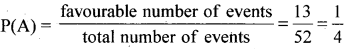(ii) P(Ā ) = P(not A) = 1 – P(A) = 1 – $$\frac{1}{4}=\frac{3}{4}$$
(iii) There are 26 black cards
∴ P(black) = 522

Part – D

Answer any SIX questions: (6 × 5 = 30)

Question 39.
Define a modulus function. Draw its graph. Also write down its domain and range.
The function f: R → R defined by f(x) = |x| for each x ∈ R is called modulus function. For each non-negative value of x, f(x) is equal to x. But for negative values of x, the value of f(x) is the negative of the value of x, i.e.,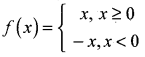Question 40.
Prove that cos2 x + cos2 $$\left(x+\frac{\pi}{3}\right)+\cos ^{2}\left(x-\frac{\pi}{3}\right)=\frac{3}{2}$$
cos2x + cos2 $$\left(x+\frac{\pi}{3}\right)+\cos ^{2}\left(x-\frac{\pi}{3}\right)$$= $$\frac { 1 }{ 2 }$$[3 + cos 2x + 2 cos 2x.cos $$\frac{2 \pi}{3}$$] = $$\frac { 1 }{ 2 }$$[3 + cos 2x + 2 cos 2x.cos$$\frac{\pi}{3}$$]
= $$\frac { 1 }{ 2 }$$[3 + cos 2x – cos 2x] = $$\frac { 3 }{ 2 }$$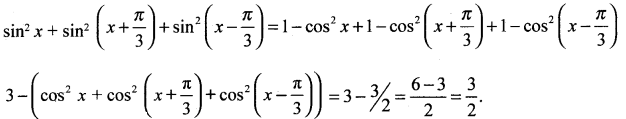Question 41.
Using mathematical induction prove that
12 + 22 + 32 + …………….. + n2 = $$\frac{n(n+1)(2 n+1)}{6}$$ ∀n ∈ N
Let P(n) : 12 + 22 + 32 + …………….. + n2 = $$\frac{n(n+1)(2 n+1)}{6}$$
step 1 : Prove that P(1) is true
when n = 1; L.H.S = 12 = 1 R.H.S = 1(1 + 1) (2 + 1)/6 = 2.3/6 = 1
∴ L.H.S = R.H.S
∴ P(1) is true

step 2: Assume that P(m) is true
i.e., 12 + 22 + 32 + ……………. + m2 = $$\frac{m(m+1)(2 m+1)}{6}$$ …(1)

step 3: Prove that P(m + 1) is true
i.e., 12 + 22 + 32 + ……….. m2 + (m + 1)2 = $$\frac{(m+1)(m+2)(2 m+3)}{6}$$
L.H.S = [12 + 22 + 32 +….. ] + (m + 1)2 = $$\frac{m(m+1)(2 m+1)}{6}$$ + (m+1)2 from (i)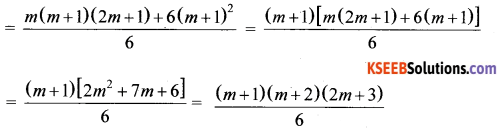= R.H.S ⇒ ∴ P(m + 1) is true
Conclusion: P(1) is true
P(m) is true ⇒ P(m + 1) is true
∴ By principle of mathematical induction, the result is true for all natural numbers ‘n’.

Question 42.
Solve the following system of inequalities graphically:
5x + 4y ≤ 20, x ≥ 1: y ≥ 2
5x + 4y ≤ 20, x ≥ 1: y ≥ 2 5x + 4y = 20,  x = 0, y = 5, y = 0, x = 4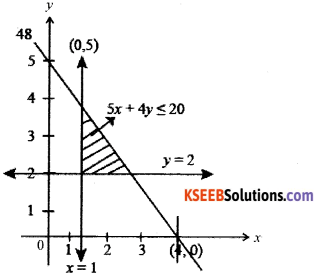Question 43.
A group consists of 5 girls and 7 boys. In how many ways can a team of 5 members be selected if the team has atleast one boy and one girl.
Number of ways of selecting
1 B and 4 G = 7C1 × 5C4 = 35
2 B and 36 = 7C2 × 5C3 = 210
3 B and 2 G = 7C3 × 5C2 = 350
4 B and 1 G = 7C4 × 5C1 = 175 (+)
Total number of selections = 770.Question 44.
state and Prove Binomial theorem for positive integer ‘n’
Statement: (a + b)n = nC0an + nc1an-1 b + nC2 an-2 b2 + nC3 an-3 b3 + …….. + nCn-1 a.bn-1 + nCnbn
Proof: By aplying principle of mathemaical induction
Let P(n) : (a + b)n = nC0an + nc1an-1b1 + ……. + nC nbn
For n = 1 p(1) = (a + b)1 = 1C0a1 + 1C1a1-1b1
[(a + b) = (a – b)] is true
For n = K(f). p(k) = (a + b)k = kC0ak + kC1ak-1b +….+ KCkbk …..(l)
is true for n = k
We shall prove that p(k + 1) is also true i.e..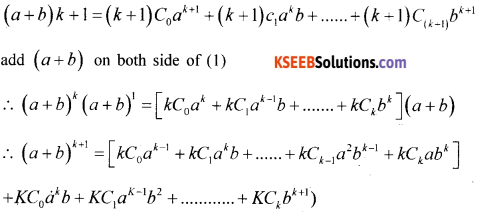Thus it has been proved that R(k + 1) is tense for p(K) is true and it is that for all positive integer n.

Question 45.
Derive the equation of a straight line in the intercept form $$\frac{x}{a}+\frac{y}{b}=1$$ and hence find the equation of the line which makes intercepts -3 and 2 on the x andy axes respectively.
Proof: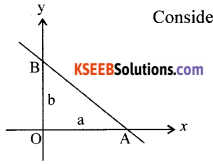∴ Equation of AB is :
Consider a line cutting x axis at A and y axis at B
x – intercept = QA = a
y – intercept = OB = b
∴ A = (a,0); B =(0, b)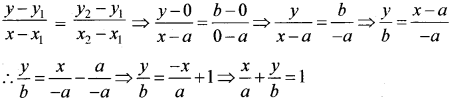Question 46.
Derive the formula for the distance between two points P(x1, y1, z1) and Q (z2, y2, z2).
Distance Formula
·Obtain the distance between the points P(x1, y1, z1,) and Q(x2, y2, z1) is given by
PQ = $$\sqrt{\left(x_{2}-x_{1}\right)^{2}+\left(y_{2}-y_{1}\right)^{2}+\left(z_{2}-z_{1}\right)^{2}}$$
Proof : Let P(x1, y1, z1,) and Q(x2, y2, z2) be the given points. Through P & Q draw PL and QM ⊥r to xy plane, meeting it in the point L and M respectively.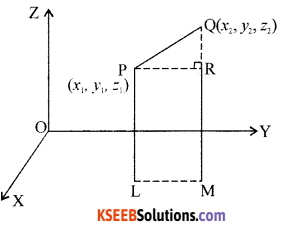In XY plane L = (x1, y1,) and M = (x2, y2)
∴ LM2 = (x2 – x1)2 + (y2 – y2)2
Through P, draw PR ⊥ QM
∴ PR = LM & QR = z2 – z1
In A PQR. PQ2 = PR2 + QR2
= LM2 + QR2 = (x2 – x1)2 + (y2 – y1)22 + (z2 =- z1,)2
∴ PQ = $$\sqrt{\left(x_{2}-x_{1}\right)^{2}+\left(y_{2}-y_{1}\right)^{2}+\left(z_{2}-z_{1}\right)^{2}}$$

Question 47.
Prove that $$\lim _{x \rightarrow 0}\left(\frac{\sin x}{x}\right)=1$$, where x is measured in radiAnswer:
Proof :- Consider a circle with centre 0 & radius ‘r’ Mark two points A & B on the circle soAt A draw a tangent to the circle. Produce OB to cut the tangent at C.
Join AB. Draw BM ⊥ OA
From the figure it is clear that
Area of ∆OAB < Area of sector OAB < sector of ∆OAC ……..(1)
Area of ∆OAB = $$\frac { 1 }{ 2 }$$ OA.BM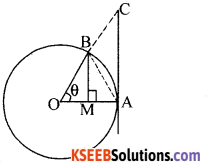[In ∆ OBM.Sin θ = $$\frac{B M}{O B}=\frac{B M}{r}$$ ⇒ BM = r Sin θ]
∴ Area of A0AB = $$\frac { 1 }{ 2 }$$ rr .sinθ = $$\frac { 1 }{ 2 }$$ r2 sinθ
Area of sector OAB = $$\frac { 1 }{ 2 }$$ r2 θ
Area of ∆OAC = $$\frac { 1 }{ 2 }$$OA.AC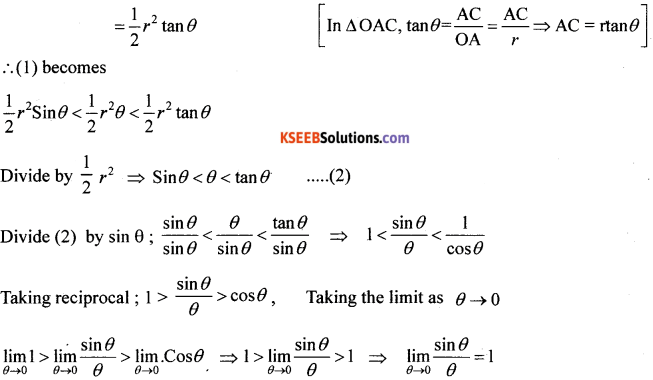Question 48.
Calculate the mean deviation about mean for the following data.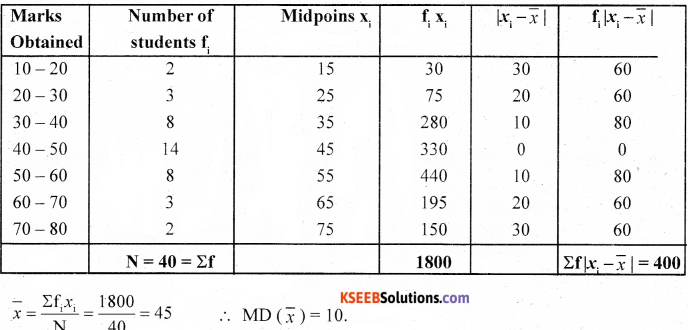Part – E

Answer any ONE question: (1 × 10 = 10)

Question 49.
(a) Prove geometrically that cos(A + B)= cos Acos B – sin Asin B.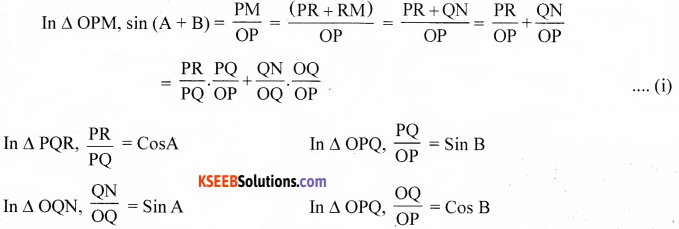∴ (i) becomes
sin (A + B) = cosA.sinB + sinA.cosB
∴ sin (A + B) = sinA cosB + cosA sinB

(b) Find the sum to n terms of the series 1 × 2 + 2 × 3 + 3 × 4 + 4 × 5 +………. (4)
Tn = (nth term of 1, 2, 3, ………..) (nth term of 2, 3, 4 ..) = n (n + 1) = n2 + n
∴ Sn = ΣTn = Σn2 + Σn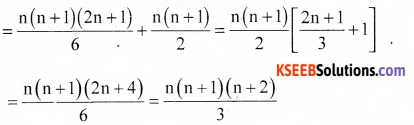Question 50.
(a) Define a parabola and derive its equation in the standard form y2 = 4ax. (6)
Parabola is the set of all points in a plane that are equidistant from a fixed line and a fixed point (not on the line) in the plane. The fixed line is called the directrix of the parabola and the fixed point F is called the focus.

A line through the focus and perpendicular to the directrix is called the axis of the parabola. The point of intersection of parabola with the axis is called the vertex of the parabola.

Let F be the focus and I the directrix. Let FM be perpendicular to the directrix and bisect FM at the point O. Produce MO to X. By the definition of parabola, the mid point O is on the parabola and is called the vertex of the parabola. Take O as origin, OX the x-axis and OY perpendicular to it as the y – axis. Let the distance from the FM directrix to the focus be 2a. Then, the coordinates of the focus are (a,0), and the equation of the directrix is x + a=0 as in Fig. Let P(x, y) be any point on the parabola such that PF = PB,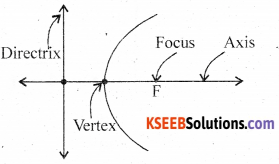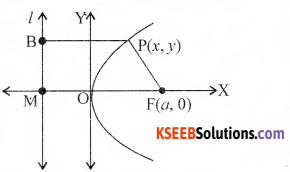where PB is perpendicular to l. The coordinates of B are (-a, y).
By the distance formula, we have
PF = $$\sqrt{(x-a)^{2}+y^{2}}$$ and PB = $$\sqrt{(x+a)^{2}}$$
since PF = PB, we have
$$\sqrt{(x-a)^{2}+y^{2}}=\sqrt{(x+a)^{2}}$$
i.e., (x – a)2 + y2 = (x + a)2
i.e., x2 – 2ax + a + y2 = x2 + 2ax + a2
i.e, y2 = 4ax (a>0).
∴ Any point on the parabola satisfies y2 = 4ax —— (1)
Conversely let P(x, y) satisfy (2)
∴ PF = $$\sqrt{(x-a)^{2}+y^{2}}=\sqrt{(x-a)^{2}+4 a x}=\sqrt{(x+a)^{2}}=P B$$. ——– (2)
and so P(x, y), lies on the parabola.
∴ From (1) and (2) we have proved that equation of parabola with vertex at the origin is y2 = 4ax.(b) Find the derivative of $$\frac{x^{5}-\cos x}{\sin x}$$ with respect to x.